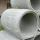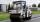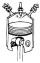Reservoir

6 m long reservoir has a diameter of 2.2 m. What is its surface area in square meters?

Result

S =  49.072 m2

Solution:Leave us a comment of example and its solution (i.e. if it is still somewhat unclear...):Be the first to comment!Next similar examples:

1. PipeHow long is the pipe with an outside diameter of 1.44 m if his coloring consumed 44 kg of color. 1 kg of color coverage is 9 m2.
2. Concrete pipeHow much will cost cover a 6 m long concrete pipe with an outer radius 1.5 m and inner radius 0.8 meters if 1 m2 paint costs 24 €.
3. Fit ballWhat is the size of the surface of Gymball (FIT - ball) with a diameter of 65 cm?
4. KitchenKitchen roller has a diameter 70 mm and width of 359 mm. How many square millimeters roll on one turn?
5. GreenhouseGarden plastic greenhouse is shaped half cylinder with a diameter of 6 m and base length 20 m. At least how many m2 of plastic is need to its cover?
6. RollerRoller has a diameter of 0.96 m and a width 169 cm. How many m2 of road level when he turns 42-times?
7. Pipe cross sectionThe pipe has an outside diameter 1100 mm and the pipe wall is 100 mm thick. Calculate the cross section of this pipe.Alaska is the largest state in the United States and has a surface area of approximately 588,000 square miles. Montana has a surface area that is approximately 25% of the surface area of Alaska. What is the approximate surface area of Montana?
9. MegapascalsWhat is the area of crosssection of the piston, if the force of 300 kN produces a pressure of 5 MPa?
10. GardenThe garden around the new majer is divided as follows: 35% vegetable, 30% fruit orchards, 10% flowers and the remaining 120 m2 are lawns. What is the total area of the garden?
11. CylinderThe cylinder surface is 859 dm2, its height is equal to the radius of the base. Calculate height of this cylinder.
12. Cylinder surface areaVolume of a cylinder whose height is equal to the radius of the base is 678.5 dm3. Calculate its surface area.
13. Shell of cylinderCalculate the content of shell the 1.6 m height cylinder with a base radius of 0.4 m.
14. Trapezium 2Trapezium has an area of 24 square cms. How many different trapeziums can be formed ?
15. Roof tilesThe roof has a trapezoidal shape with bases of 15 m and 10 m, height of roof is 4 meters. How many tiles will need if on 1 m2 should be used 8 tiles?
16. TrapeziumThe area of trapezium is 35cm2 find its altitude if the bases are 6cm and 8cm.
17. RhombusPQRS is a rhombus. Given that PQ=3 cm & height of rhombus is given 2 cm. Calculate its area.